Previous: 2.2.2.1 Even Moments Up: 2.2.2 Hierarchy of Moment Next: 2.2.2.3 Non-Parabolicity Corrections

### 2.2.2.2 Odd Moments

For the odd moments we obtain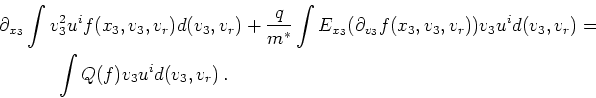(2.35)

For the first term we note that by the assumption of isotropy of the symmetric part we have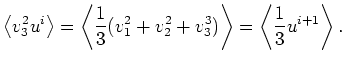(2.36)

By using partial integration on the second term we obtain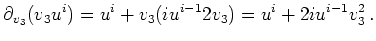(2.37)

With symmetric isotropy we get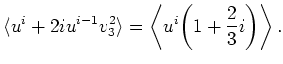(2.38)

In summary we get the following set of equations: For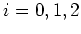and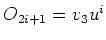: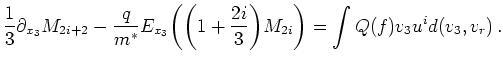(2.39)

The derivation does not need the full isotropy condition for its validity. Instead, we only need two reduction conditions: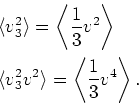(2.40)

A third reduction condition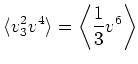(2.41)

is not needed if the sixth moment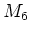is redefined as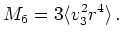(2.42)

So by using the isotropy condition we can eliminate the moment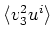. For the validity of Equation 2.36 the isotropy assumption is a sufficient, but not a necessary condition. A simple counter example is a function of the form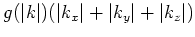. However, this form does not fulfill cylindrical symmetry. Examples which fulfill cylindrical symmetry can be constructed too, but one has to consider multivariate polynomials up to sixth order for a proof.